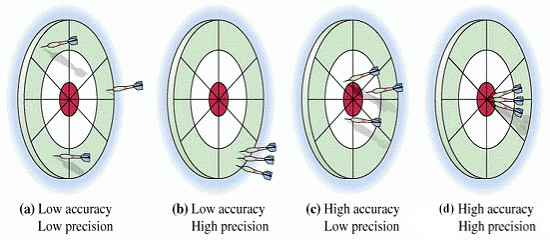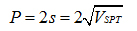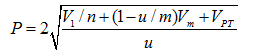Call us: 86-731-84012074Brief Discussion on Sampling Precision

October.31

Written by CKIC Product Manager, Mr. Li Jingya

1. Precision and Accuracy
a. Precision
Precision refers to consistency in the independent test results under prescribed conditions.

b. Accuracy
Accuracy refers to the consistency between the test result and the measured true value or the conventional truth value.

The figure below clearly shows the relationship between precision and accuracy.2. Sampling Precision
Sampling precision refers to the limiting value of the differences between the result of a single sampling measurement and the mean value of the same coal (same source, same property) by countless sampling (at 95% probability).

For example, carry out countless sampling for a certain batch of coal.

When the average value of ash (Ad) is25% and the standard deviation (S) is 1% for each coal sample, the sampling precision is P = 2%, and there is 95% probability to control the measured values of single sampling ash within the range of (23 to 27)%. The smaller the sampling precision is, the smaller the sampling random error is, and the smaller the fluctuation of the lot testing result.

3. Determination of Sampling Precision
a. Sampling precision is actually the allowed random error that our sampling method can reaches, which can be accepted by all the trading parties.
The following factors are required to be considered for the determination of sampling precision:
① Acceptable Sampling Precision Values for All the Trade Parties;
② Operability of Sampling Method;
③ Uneven Degree of Coal Quality in China (Variation of Coal);

b. Precision Value

Sampling Precision Under GB 475 Basic Sampling Scheme

 Raw Coal, Screened Coal Cleaned Coal Other Washed Coal (Middling Coal) Ad≤20% Ad>20% ±1% ±1.5% ±1/10×Ash (≥ ±1%) ±2%

GB/T 19494.1 Total Precision of Coal Mechanization Sampling

 Coal Type Precision Ad (%) Cleaned Coal ±0.8 Other Coal ±0.1×Ad ≤1.6

c. Expected Precision and the Least Allowable Precision

In fact, the sampling precision is divided into the expected precision and the least allowable precision. The expected precision represents the sampling precision expected for the sampling scheme designed for the coal sample. The least allowable precision represents the least sampling precision that can be received by the sampling scheme designed for the coal sample. The precision value of the sample specified in GB 475 is actually the least allowable precision value. As the expected precision, the precision trading parties required is less than the precision specified in GB 475.We used to regard the expected precision value and the minimum allowable precision as one same value. They both use the sampling precision values specified in GB 475.

4. Calculation Formula
a. Basis FormulaWhere: S
— Overall Standard Deviation of Sample
VSPT
Total Variance of Production

b. Precision Formula under the Discontinuous SamplingWhere: m
— Total Number of sub-lot.
u — Number of Actual Sub-lot.
V1— Primary Sample Variance.
V— Sub-lot Variance
VPT — Sample Preparation and Analysis Variance

c. Precision Formula under the Continuous Sampling (u=m)d. When a batch of coal is used as one sub-lot(m=1), the precision formula is as shown as below:5. Examples
Presume that one power plant receives a lot of raw coal shipped by train. There are 50 carriages in total, 65t for each carriage. According to past experience, the nominal top size of coal is 80mmand the ash content is 25% ~ 35%.

A. Develop the coal sampling program according to the sampling requirements of the GB 475-2008.
1) Make the coal as one sub-lot.

a. The coal is taken as one sub-lot with an expected sampling precision of ± 2.0% (on dry basis)
b. The minimum increment is calculated as below:c. The particle size is 80mm, and the minimum mass of total sample is 565kg.
d. The average increment mass is: m'=mg/n=565/109=5.2kg
e. The minimum mass of the increment: ma=0.06×80=4.8kg.
f. Since m'>ma, take 5.2 kg coal as the increment.

Therefore, according to the basic sampling program of GB475-2008, make the coal as one sub-lot, and take 109 samples in total. The average mass of each sample is 5.2kg, and the expected sampling precision can reach up to ± 2.0% (On dry basis).

2) Take the coal as two sub-lots.

a. The coal is taken as two sub-lots with an expected sampling precision of ± 2.0% each(on dry basis). This expected sampling precision for this coal batch is±1.4%（On dry basis
b. The minimum increment is calculated as below:c. The particle size is 80mm, and the minimum mass of total sample is 565kg.
d. The average increment mass is：m'=mg/n=565/77=7.4kg.
e. The minimum mass of the increment: ma=0.06×80=4.8kg.
f. Since m'>ma, take 7.4 kg coal as the increment.

Therefore, according to the basic sampling program of GB475-2008, make the coal as two sub-lot, and take 77 samples in total. The average mass of each sample is 7.4kg, and the expected sampling precision can reach up to ± 1.4% (On dry basis).

B. Develop the coal sampling program according to the sampling requirements of the GB19494.1-2004.
1) Make the coal lot as one sub-lot.
a. The coal is taken as one sub-lot with an expected sampling precision of ± 1.6% (on dry basis)
b. The minimum increment is calculated as below:c. The particle size is 80mm, and the minimum mass of total sample is 565kg.
d. The average increment mass is：m'=mg/n=565/82=6.9kg
e. The minimum mass of the increment: ma=0.001×802=6.4kg
f. Since m'>ma, take 6.9 kg coal as the increment.

Therefore, according to the basic sampling program of GB19494.1-2004, make the coal as one sub-lot, and take 82 samples in total. The average mass of each sample is 6.9 kg, and the expected sampling precision can reach up to ± 1.6% (On dry basis ).

2) Make the coal as two sub-lots.

g. The coal is taken as two sub-lots with an expected sampling precision of ± 1.6% each (on dry basis). This expected sampling precision for this coal batch is ±1.1% (On dry basis)
h. The minimum increment is calculated as below:i. The particle size is 80mm, and the minimum mass of total sample is 565kg.
j. The average increment mass is: m'=mg/28=565/58=9.8kg.
k. The minimum mass of the increment: ma=0.001×802=6.4kg.
l. Since m'>ma, take9.8Kgcoal as the increment.

Therefore, according to the basic sampling program of GB19494.1-2004, make the coal as two sub-lots, and take 58 samples in total. The average mass of each sample is 9.8kg, and the expected sampling precision can reach up to ± 1.1% (On dry basis ).

By comparing the results of data analysis, it can be found that the precision of mechanical sampling is better than that of manual sampling. No matter it is mechanical sampling or manual sampling, if there are more sub-lots, the precision will be higher. At present, mechanical sampling almost entirely replaces manual sampling.